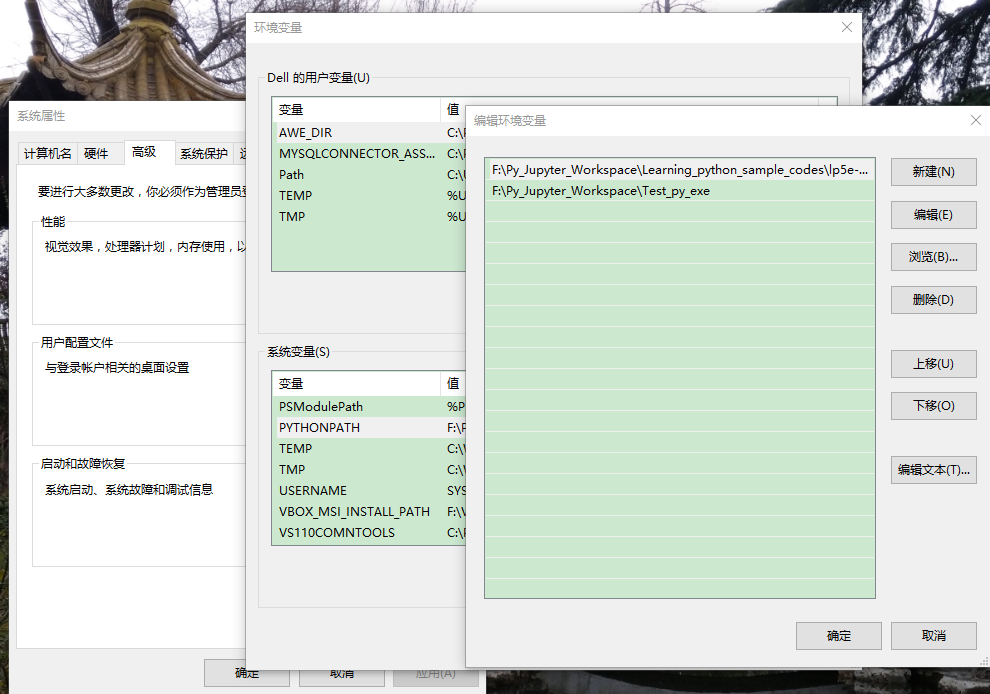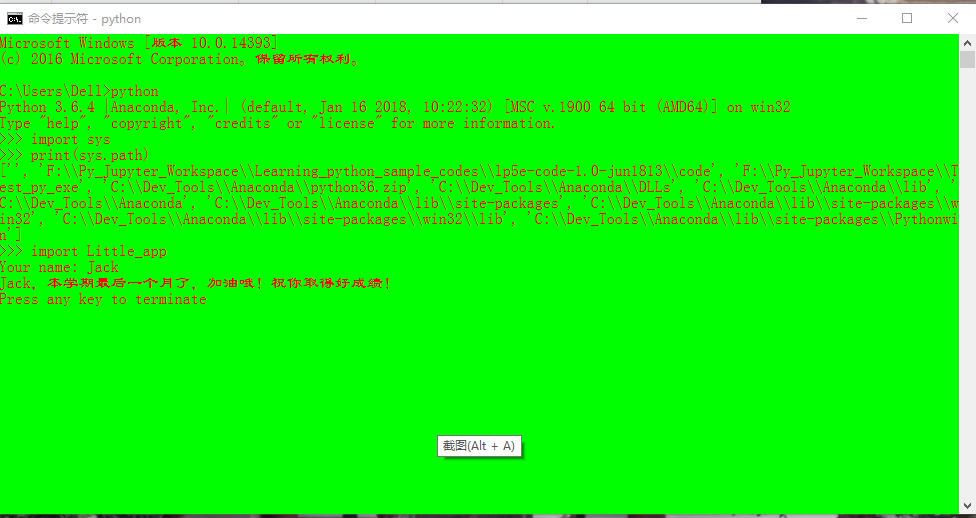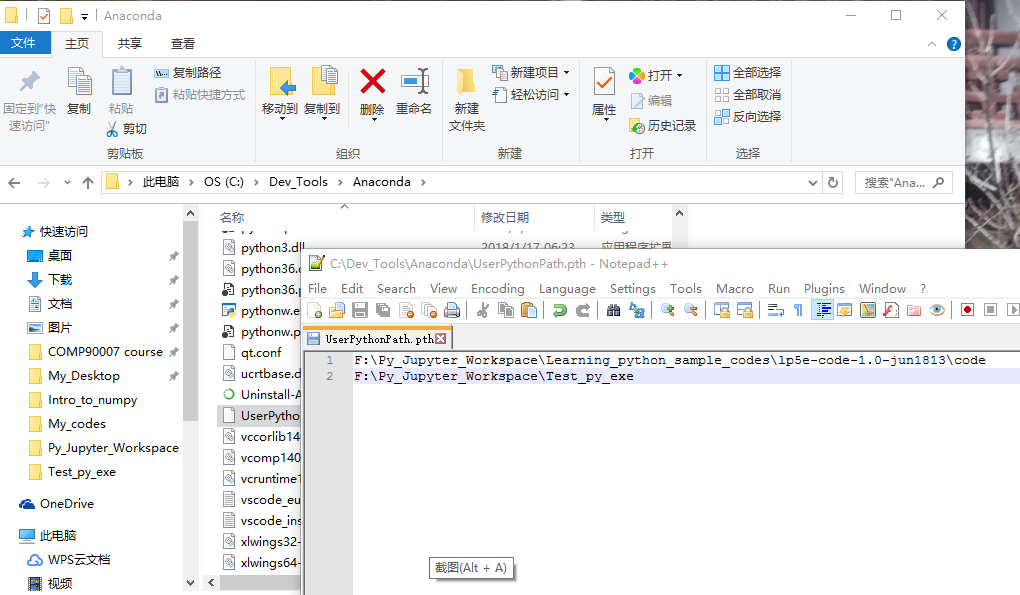## 1. Python普通模块制作和导入：

``````import somemodule
from somemodule import somefunction
from somemodule import *
``````

• 第一步，打开python IDE，编写Hello, world程序，输入代码：
``````print "Hello, world!"
def hello():
print "Hello, world!"
``````
• 第二步，保存至任意路径后进行调试，文件名为Hellopy。本例保存在：E:\Program_Dirs\Py_workspace\Py_Study

（本步骤为导入任何自制或第三方库的办法）确认程序调试完成后，运行以下代码：

``````>>> import sys
>>> sys.path.append('E:/Program_Dirs/Py_workspace/Py_Study')
>>> import Hellopy
Hello, world!
>>> Hellopy.hello()
Hello, world!
``````

• 第三步，导入后，文件所在目录下会产生一个.pyc文件，会有利于python今后快速进行调用，用户可以选择删除或不删除。（参考资料1第210页）## 2. Python系统模块制作和导入：

``````>>> import sys, pprint
>>> pprint.pprint(sys.path)
['',
'D:\\Develop_Tools\\Py27\\Lib\\idlelib',
'C:\\Windows\\system32\\python27.zip',
'D:\\Develop_Tools\\Py27\\DLLs',
'D:\\Develop_Tools\\Py27\\lib',
'D:\\Develop_Tools\\Py27\\lib\\plat-win',
'D:\\Develop_Tools\\Py27\\lib\\lib-tk',
'D:\\Develop_Tools\\Py27',
'D:\\Develop_Tools\\Py27\\lib\\site-packages']
>>>
``````

``````>>> import Hellopy
>>> Hellopy.hello()
Hello, world!
``````

#### 1、通过设置环境变量PYTHONPATH扩展系统路径#### 2、通过制作.pth文件扩展系统路径### 3. Python的包：

Python中，包事实上是一个路径，该路径含有文件__init__.py。init.py文件中含有的内容即包的内容。比如有个被放在库函数搜索路径中的包叫做constants，其中有句代码PI = 3.14，则可以通过如下代码查看这个常量：

``````>>> import constants
>>> constants.PI
3.14
``````

``````# (1) Imports the drawing package:
import drawing
# (2) Imports the colors module (a file of drawing):
import drawing.colors
# (3) Imports the shapes module
# (also a file of drawing):
from drawing import shapes
``````

python的每个模块即使一个python文件，从面向对象的角度来看，这文件也是一个命名空间。（namespace）。使用dir方法可以快速查看一个模块中所有的属性（attribute），包括这个模块中的各个类、方法、函数以及变量。

``````>>> a = (n for n in dir(copy) if n.startswith('_'))
>>> print list(a)
['_EmptyClass', '__all__', '__builtins__', '__doc__', '__file__', '__name__', '__package__', '_copy_dispatch', '_copy_immutable', '_copy_inst', '_copy_with_constructor', '_copy_with_copy_method', '_deepcopy_atomic', '_deepcopy_dict', '_deepcopy_dispatch', '_deepcopy_inst', '_deepcopy_list', '_deepcopy_method', '_deepcopy_tuple', '_keep_alive', '_reconstruct', '_test']
``````

``````Model.__all__
``````

``````from Model import *
``````

``````>>> copy.__all__
['Error', 'copy', 'deepcopy']
``````

``````from copy import PyStringMap
``````

Python中，使用help(model)方法可以查看model模块的相关信息。而使用model.__doc__则可以查看对于该模块的文档说明。更多的python文档，请参考：https://www.python.org/doc/

Python中，使用__file__可以查看模块所在路径，例如：

``````>>> import copy
>>> print copy.__file__
D:\Python27_13\lib\copy.pyc
``````

#### 4. Python几个常用模块简介：

##### sys 模块：
• argv：获取控制行输入的若干参数
• exit([arg])：退出当前程序
• modules：sys.modules查询当前系统模块包含的变量或类的路径
• path：sys.path查询当前模块所处路径，以列表形式返回
• platform：sys.platform查询当前解释器运行的平台
• stdin, stdout, stderr：常见输入输出流

``````#Reverseargs.py
import sys
args = sys.argv[1: ]
args.reverse()
print ' '.join(args)

python Reverseargs.py This is a test

test a is This
``````

python os模块收录一些常用的在python中进行系统操作的方法，详细资料见参考资料1第221-223页。例如，在python shell中打开QQ浏览器做法如下：

``````>>> os.startfile(r"D:\Internet_Tools\QQ_Browser\QQBrowser\QQBrowser.exe")
``````

``````>>> import webbrowser
>>> webbrowser.open('http://www.python.org')
True
``````
##### time 模块：
• asctime([tuple])：显示表示当地时间的字符串，可选参数为localtime或gmtime，默认为当地时间。
• sleep(sec)：在sec秒之内不做任何事情。
• time()：从 UNIX纪元到当前时间的浮点秒数。
• strptime(string[, format]))：将字符形式的时间转化为时间元组。

time模块使用举例1：asctime使用

``````>>> import time
>>> time.asctime(time.gmtime())
'Tue Mar 28 04:04:20 2017'
>>> time.asctime(time.localtime())
'Tue Mar 28 12:04:59 2017'
>>> time.asctime()
'Tue Mar 28 12:05:06 2017'
``````

time模块使用举例2：strptime使用

``````>>> time.strptime("28 Mar 17", "%d %b %y")
time.struct_time(tm_year=2017, tm_mon=3, tm_mday=28, tm_hour=0, tm_min=0, tm_sec=0, tm_wday=1, tm_yday=87, tm_isdst=-1)
``````

##### random 模块：
• random：返回区间[0.0, 1.0)之间的实数。
• getrandbits(n)：返回从0到2^n的n位2进制数。
• uniform(a, b)：返回区间[a, b]内的一个实数。
• randrange([start], stop, [step]) ：返回从start到stop区间内的一个随机实数。其中start参数若不选默认为0。
• choice(seq)：返回一列元素中的任意一个
• shuffle(seq[, random]) ：打乱一个序列
• sample(seq, n)：从seq序列中取出n个样本

random模块使用举例：

``````from random import *
from time import *

#Generate a random time of this year:
date1 = (2017, 1, 1, 0, 0, 0, -1, -1, -1)
date2 = (2018, 1, 1, 0, 0, 0, -1, -1, -1)
time1 = mktime(date1)
time2 = mktime(date2)
rand_time = uniform(time1, time2)
print asctime(localtime(rand_time))

Sun Apr 09 22:16:14 2017
``````
##### shelve模块：

``````# database.py
import sys, shelve

def store_person(db):
"""
Query user for data and store it in the shelf object
"""
pid = raw_input('Enter unique ID number: ')
code omitted. """
#Actually expand the shelve obj here.
db[pid] = person

def lookup_person(db):
"""
Query user for ID and desired field, and fetch the corresponding data from
the shelf object
"""
pid = raw_input('Enter ID number: ')
#Example of looking person obj according to pid and field.
print field.capitalize() + ':'+ db[pid][field]

def main():
# You may want to change this name
database = shelve.open('E:\\Program_Dirs\\Py_workspace\\Py_Study\\shelveDB.txt')
try:
while True:
cmd = enter_command()
if cmd == 'store':
store_person(database)
elif cmd == 'lookup':
lookup_person(database)
#unimportant code, omitted.

finally:
"""Do not forget to close in order to make
any possible update valid."""
database.close()

if __name__ == '__main__': main()
``````
##### re模块：

re模块为python的正则表达式模块，常用函数有compile, search, match, split, findall, sub, escape, group, start, end以及span等。主要用于python中正则表达式的处理操作。举例如：

``````>>> m = re.match(r'www\.(.*)\..{3}', 'www.python.org')
>>> m.group(1)
'python'
>>> m.start(1)
4
>>> m.end(1)
10
>>> m.span(1)
(4, 10)
``````

1. Magnus Lie Hetland, Beginning Python, From Novice to Professional, Second Edition, in Apress
2. 彭博，Python正则表达式指南，URL：https://my.oschina.net/dtec/blog/40947
3. Python lib, random module, URL: https://docs.python.org/2/library/random.html#random.SystemRandom
4. Python lib, time module, URL: https://docs.python.org/2/library/time.html#module-time